Courses

# RD Sharma Solutions - Ex-5.4 & Ex-5.5, Operations On Rational Numbers, Class 7, Math Class 7 Notes | EduRev

## Class 7 : RD Sharma Solutions - Ex-5.4 & Ex-5.5, Operations On Rational Numbers, Class 7, Math Class 7 Notes | EduRev

The document RD Sharma Solutions - Ex-5.4 & Ex-5.5, Operations On Rational Numbers, Class 7, Math Class 7 Notes | EduRev is a part of the Class 7 Course RD Sharma Solutions for Class 7 Mathematics.
All you need of Class 7 at this link: Class 7

Ex-5.4

Q1. Divide:

Solution.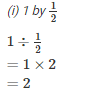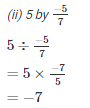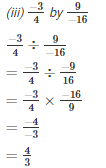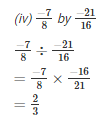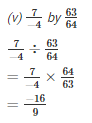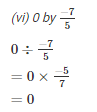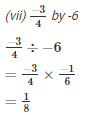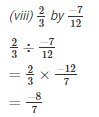Q2. Find the value and express as a rational number in standard form:

Solution.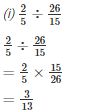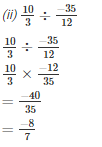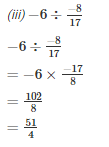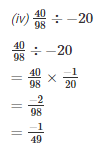Q3. The product of two rational numbers is 15. If one of the numbers is -10, find the other.

Solution.

Let the number to be found be x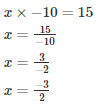Hence the number is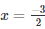Q4. The product of two rational numbers is −8/9. If one of the numbers is −4/15, find the other.

Solution.

Let the number to be found be x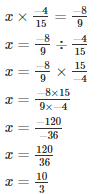Hence the number is x = 10/3

Q5. By what number should we multiply −1/6 so that the product may be −23/9?

Solution.

Let the number to be found be x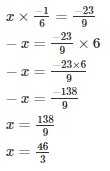Hence the number is x = 46/3

Q6. By what number should we multiply −15/28 so that the product may be −5/7?

Solution.

Let the number to be found be x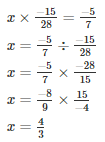Hence the number is x = 4/3

Q7. By what number should we multiply −8/13 so that the product may be 24?

Solution.

Let the number to be found be x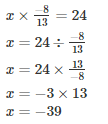Hence the number is x=−39

Q8. By what number should −3/4 be multiplied in order to produce −2/3?

Solution.

Let the number to be found be x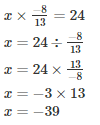Hence the number is x = −39

Q9. Find (x + y)÷(x —y), if

Solution.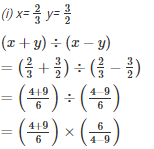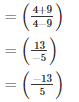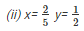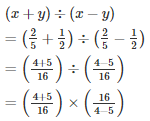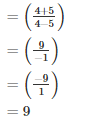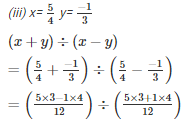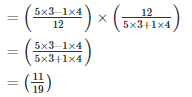Q10. The cost of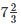metres of rope is Rs.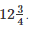Find its cost per metre.

Solution.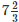metres of rope cost= Rs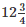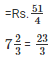Cost per metre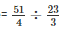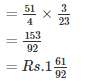Q11. The cost of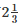metres of cloth is Rs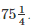Find the cost of cloth per metre.

Solution.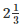metres of rope cost =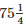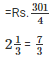Cost per metre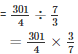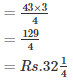Q12. By what number should −33/16 be divided to get  −11/4?

Solution.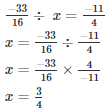The number is x = 3/4

Q13. Divide the sum of −13/5 and 12/7 by the product of −31/7 and −1/2

Solution.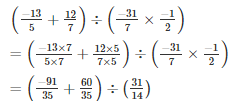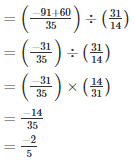Q14. Divide the sum of 65/12 and 8/3 by their difference.

Solution.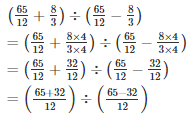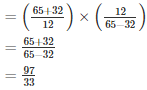Q15. If 24 trousers of equal size can be prepared in 54 metres of cloth, what length of cloth is required for each trouser?

Solution.

Length of cloth required for each trouser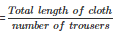= 54/24

= 94metres

9/4mmetres of cloth is required to make each trouser

Ex-5.5

Q1. Find six rational numbers between −4/8 and 3/8

We know that

-4,-3,-2,-1,0,1,2,3

Therefore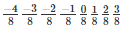Hence 6 rational numbers between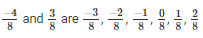Q2. Find 10 rational numbers between 7/13 and −4/13

We know that

76543210-1-2-3-4

Therefore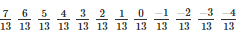Hence the 10 rational numbers between 7/13 and −4/13 are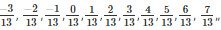Q3. State true or false:

(i) Between any two distinct integers there is always an integer.

FALSE

(ii) Between any two distinct rational numbers there is always a rational number.

TRUE

(iii) Between any two distinct rational numbers there are infinitely many rational numbers.

TRUE

Offer running on EduRev: Apply code STAYHOME200 to get INR 200 off on our premium plan EduRev Infinity!

97 docs

,

,

,

,

,

,

,

,

,

,

,

,

,

,

,

,

,

,

,

,

,

,

,

,

,

,

,

,

,

,

;